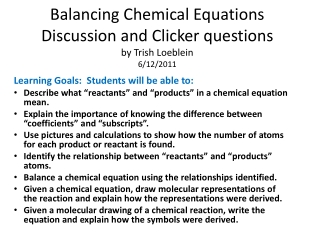DownloadDownload PresentationBalancing Chemical Equations Discussion and Clicker questions by Trish Loeblein 6/12/2011

# Balancing Chemical Equations Discussion and Clicker questions by Trish Loeblein 6/12/2011

Télécharger la présentation## Balancing Chemical Equations Discussion and Clicker questions by Trish Loeblein 6/12/2011

- - - - - - - - - - - - - - - - - - - - - - - - - - - E N D - - - - - - - - - - - - - - - - - - - - - - - - - - -
##### Presentation Transcript

1. Balancing Chemical Equations Discussion and Clicker questionsby Trish Loeblein6/12/2011 Learning Goals: Students will be able to: Describe what “reactants” and “products” in a chemical equation mean. Explain the importance of knowing the difference between “coefficients” and “subscripts”. Use pictures and calculations to show how the number of atoms for each product or reactant is found. Identify the relationship between “reactants” and “products” atoms. Balance a chemical equation using the relationships identified. Given a chemical equation, draw molecular representations of the reaction and explain how the representations were derived. Given a molecular drawing of a chemical reaction, write the equation and explain how the symbols were derived.

2. 1. What would you do to balance this reaction? • Double the coefficient of N2(2 N2 ) • Multiply coefficient of H2 by 3 (3 H2 ) • Multiply subscripts of H2 by 3 ( H6) • Double the subscripts for NH3(N2H6) • Double the coefficient of NH3 (2NH3)

3. 2. Which visual cues can you use on a test to see if your equation is balanced or not?

4. 3. Which chemicals are reactants? • HN3 and O2 • O2 and H2O • N2 and H2O • NH3 and N2

5. 4. Which best describes the products of a chemical equation? • Chemicals before the reaction starts • Chemicals after the reaction ends • Chemicals on the left of the arrow • Chemicals on the right of the arrow

6. 5. Which are the products of this chemical equation? • a • b • F2 and H2O • OF2 and HF • More than 2 answers

7. Which best describes the products of a chemical equation? An author of a test or text may chose to write this reaction: Lesson learned: Don’t try to memorize reactions, analyze each one that is given.

8. 6.Is this reaction balanced? • Yes • No, there needs to be fewer red on the reactant side. • No, there needs to be more red on the product side. • No, for another reason.